# 564 and Level 6

Consecutive primes 281 and 283 add up to 564.

564 is made from three consecutive numbers so it can be evenly divided by 3. If the middle number is divisible by 3, then a number made from three consecutive numbers will also be divisible by 9. Is 564 divisible by 9? Why or why not?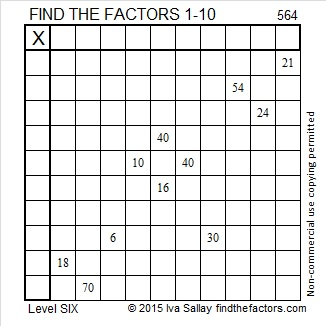Print the puzzles or type the solution on this excel file: 10 Factors 2015-07-20

—————————————————————————————————

• 564 is a composite number.
• Prime factorization: 564 = 2 x 2 x 3 x 47, which can be written 564 = (2^2) x 3 x 47
• The exponents in the prime factorization are 2, 1, and 1. Adding one to each and multiplying we get (2 + 1)(1 + 1)(1 + 1) = 3 x 2 x 2 = 12. Therefore 564 has exactly 12 factors.
• Factors of 564: 1, 2, 3, 4, 6, 12, 47, 94, 141, 188, 282, 564
• Factor pairs: 564 = 1 x 564, 2 x 282, 3 x 188, 4 x 141, 6 x 94, or 12 x 47
• Taking the factor pair with the largest square number factor, we get √564 = (√4)(√141) = 2√141 ≈ 23.74868—————————————————————————————————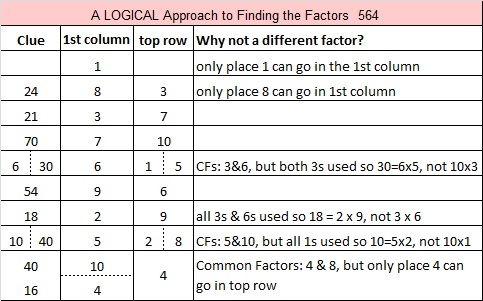# 563 and Level 5

563 is prime, and it is the sum of all the prime numbers from 5 to 67.Print the puzzles or type the solution on this excel file: 10 Factors 2015-07-20

—————————————————————————————————

• 563 is a prime number.
• Prime factorization: 563 is prime.
• The exponent of prime number 563 is 1. Adding 1 to that exponent we get (1 + 1) = 2. Therefore 563 has exactly 2 factors.
• Factors of 563: 1, 563
• Factor pairs: 563 = 1 x 563
• 563 has no square factors that allow its square root to be simplified. √563 ≈ 23.727621How do we know that 563 is a prime number? If 563 were not a prime number, then it would be divisible by at least one prime number less than or equal to √563 ≈ 23.7. Since 563 cannot be divided evenly by 2, 3, 5, 7, 11, 13, 17, 19, or 23, we know that 563 is a prime number.

—————————————————————————————————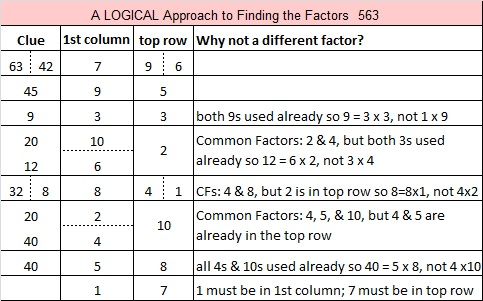# 562 and Level 4

562 is the sum of all the prime numbers from 23 to 71.

562 is also the hypotenuse of the Pythagorean triple 320-462-562. Which factor of 562 is the greatest common factor of those three numbers?Print the puzzles or type the solution on this excel file: 10 Factors 2015-07-20

—————————————————————————————————

• 562 is a composite number.
• Prime factorization: 562 = 2 x 281
• The exponents in the prime factorization are 1 and 1. Adding one to each and multiplying we get (1 + 1)(1 + 1) = 2 x 2 = 4. Therefore 562 has exactly 4 factors.
• Factors of 562: 1, 2, 281, 562
• Factor pairs: 562 = 1 x 562 or 2 x 281
• 562 has no square factors that allow its square root to be simplified. √562 ≈ 23.7065—————————————————————————————————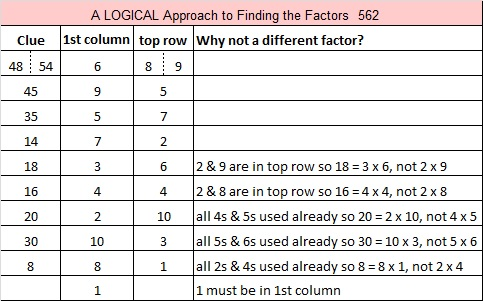# 560 and Level 3

560 is the hypotenuse of the Pythagorean triple 336-448-560. What is the greatest common factor of those three numbers?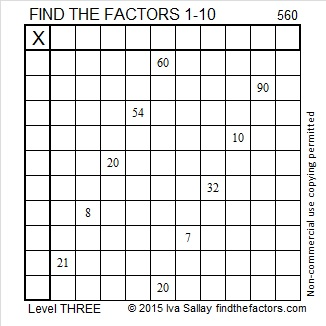Print the puzzles or type the solution on this excel file: 10 Factors 2015-07-20

—————————————————————————————————

• 560 is a composite number.
• Prime factorization: 560 = 2 x 2 x 2 x 2 x 5 x 7, which can be written 560 = (2^4) x 5 x 7
• The exponents in the prime factorization are 4, 1 and 1. Adding one to each and multiplying we get (4 + 1)(1 + 1)(1 + 1) = 5 x 2 x 2 = 20. Therefore 560 has exactly 20 factors.
• Factors of 560: 1, 2, 4, 5, 7, 8, 10, 14, 16, 20, 28, 35, 40, 56, 70, 80, 112, 140, 280, 560
• Factor pairs: 560 = 1 x 560, 2 x 280, 4 x 140, 5 x 112, 7 x 80, 8 x 70, 10 x 56, 14 x 40, 16 x 35 or 20 x 28
• Taking the factor pair with the largest square number factor, we get √560 = (√16)(√35) = 4√35 ≈ 23.664319—————————————————————————————————

A Logical Approach to solve a FIND THE FACTORS puzzle: Find the column or row with two clues and find their common factor. Write the corresponding factors in the factor column (1st column) and factor row (top row).  Because this is a level three puzzle, you have now written a factor at the top of the factor column. Continue to work from the top of the factor column to the bottom, finding factors and filling in the factor column and the factor row one cell at a time as you go.# 559 and Level 2

All the prime numbers from 67 to 97 add up to 559, and all the prime numbers from 103 to 127 also add up to 559.

559 is also the hypotenuse of Pythagorean triple 215-516-559. What is the greatest common factor of those three numbers?Print the puzzles or type the solution on this excel file: 10 Factors 2015-07-20

—————————————————————————————————

• 559 is a composite number.
• Prime factorization: 559 = 13 x 43
• The exponents in the prime factorization are 1 and 1. Adding one to each and multiplying we get (1 + 1)(1 + 1) = 2 x 2 = 4. Therefore 559 has exactly 4 factors.
• Factors of 559: 1, 13, 43, 559
• Factor pairs: 559 = 1 x 559 or 13 x 43
• 559 has no square factors that allow its square root to be simplified. √559 ≈ 23.64318—————————————————————————————————# 553 and Level 3

79 is a factor of 553. If you start at 79 and go backwards adding all the prime numbers from 79 to 43, the sum will be 553. Do you know those prime numbers forwards and backwards well enough to do that?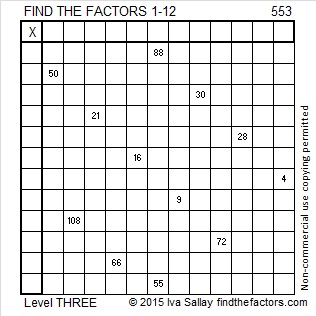Print the puzzles or type the solution on this excel file: 12 Factors 2015-07-13

—————————————————————————————————

• 553 is a composite number.
• Prime factorization: 553 = 7 x 79
• The exponents in the prime factorization are 1 and 1. Adding one to each and multiplying we get (1 + 1)(1 + 1) = 2 x 2 = 4. Therefore 553 has exactly 4 factors.
• Factors of 553: 1, 7, 79, 553
• Factor pairs: 553 = 1 x 553 or 7 x 79
• 553 has no square factors that allow its square root to be simplified. √553 ≈ 23.51595—————————————————————————————————

A Logical Approach to solve a FIND THE FACTORS puzzle: Find the column or row with two clues and find their common factor. Write the corresponding factors in the factor column (1st column) and factor row (top row).  Because this is a level three puzzle, you have now written a factor at the top of the factor column. Continue to work from the top of the factor column to the bottom, finding factors and filling in the factor column and the factor row one cell at a time as you go.# Level 2 and Simplifying √552

552 is the sum of all the prime numbers from 37 to 73.

It is also the sum of all the prime numbers from 79 to 103.

That means if you add up all the prime numbers from 37 to 103, you’ll get 1104 which is 2 x 552.

Can you list all those prime numbers?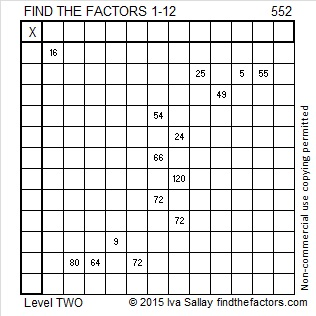Print the puzzles or type the solution on this excel file: 12 Factors 2015-07-13

—————————————————————————————————

I’ve modified the cake method to help me find √552.  My philosophy is that there is no reason to completely break up 552 to find its square root when we’re just going to have to put it mostly back together again to give the final answer. About 83% of numbers whose square roots can be reduced are divisible by 4 and/or 9. It makes sense to check to see if 552 is divisible by either of those numbers first.

552 can be evenly divided by 4 because its last two digits are divisible by 4. That means √552 can be reduced. Let’s find 552 ÷ 4.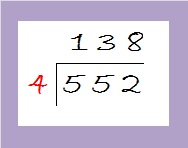The quotient is 138 which is even but not divisible by 4. Let’s check to see if it is divisible by nine: 1 + 3 + 8 = 12. It is divisible by 3, but not by 9.

Since 138 is even and divisible by 3, it is divisible by 6. It is easier to divide by 6 once than it is to divide first by 2 and then by 3. Dividing by 6 makes our division cake look like this: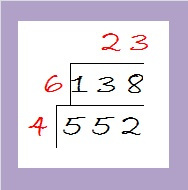Since 23 is a prime number, we are done with the division process. Taking the square root of everything on the outside of the cake we get √552 = (√4)(√6)(√23) = (√4)(√138) = 2√138.

—————————————————————————————————

• 552 is a composite number.
• Prime factorization: 552 = 2 x 2 x 2 x 3 x 23, which can be written 552 = (2^3) x 3 x 23
• The exponents in the prime factorization are 3, 1, and 1. Adding one to each and multiplying we get (3 + 1)(1 + 1)(1 + 1) = 4 x 2 x 2 = 16. Therefore 552 has exactly 16 factors.
• Factors of 552: 1, 2, 3, 4, 6, 8, 12, 23, 24, 46, 69, 92, 138, 184, 276, 552
• Factor pairs: 552 = 1 x 552, 2 x 276, 3 x 184, 4 x 138, 6 x 92, 8 x 69, 12 x 46, or 23 x 24
• Taking the factor pair with the largest square number factor, we get √552 = (√4)(√138) = 2√138 ≈ 23.49468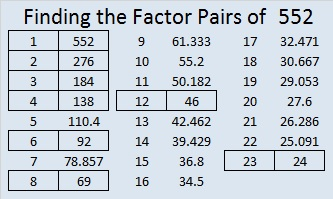—————————————————————————————————# 551 and Level 1

551 is the sum of all the prime numbers from 11 to 67. It also equals this sum of consecutive primes: 179 + 181 + 191.

551 is the hypotenuse of the Pythagorean triple 380-399-551. What is the greatest common factor of those three numbers? Hint: It’s one of the factors of 551 listed below the puzzle.Print the puzzles or type the solution on this excel file: 12 Factors 2015-07-13

—————————————————————————————————

• 551 is a composite number.
• Prime factorization: 551 = 19 x 29
• The exponents in the prime factorization are 1 and 1. Adding one to each and multiplying we get (1 + 1)(1 + 1) = 2 x 2 = 4. Therefore 551 has exactly 4 factors.
• Factors of 551: 1, 19, 29, 551
• Factor pairs: 551 = 1 x 551 or 19 x 29
• 551 has no square factors that allow its square root to be simplified. √551 ≈ 23.453389—————————————————————————————————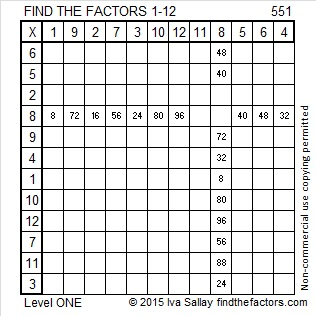# 549 and Level 6

549 is the hypotenuse of the Pythagorean triple 99-540-549. What is the greatest common factor of those three numbers?Print the puzzles or type the solution on this excel file: 10 Factors 2015-07-06

—————————————————————————————————

• 549 is a composite number.
• Prime factorization: 549 = 3 x 3 x 61, which can be written 549 = (3^2) x 61
• The exponents in the prime factorization are 2 and 1. Adding one to each and multiplying we get (2 + 1)(1 + 1) = 3 x 2  = 6. Therefore 549 has exactly 6 factors.
• Factors of 549: 1, 3, 9, 61, 183, 549
• Factor pairs: 549 = 1 x 549, 3 x 183, or 9 x 61
• Taking the factor pair with the largest square number factor, we get √549 = (√9)(√61) = 3√61 ≈ 23.430749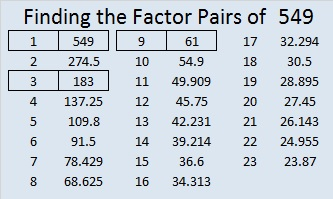—————————————————————————————————# 548 and Level 5

548 is the hypotenuse of the Pythagorean triple 352-420-548. What is the greatest common factor of those three numbers?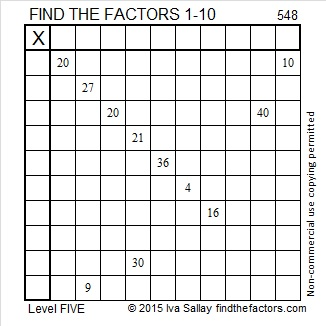Print the puzzles or type the solution on this excel file: 10 Factors 2015-07-06

—————————————————————————————————

• 548 is a composite number.
• Prime factorization: 548 = 2 x 2 x 137, which can be written 548 = (2^2) x 137
• The exponents in the prime factorization are 2 and 1. Adding one to each and multiplying we get (2 + 1)(1 + 1) = 3 x 2  = 6. Therefore 548 has exactly 6 factors.
• Factors of 548: 1, 2, 4, 137, 274, 548
• Factor pairs: 548 = 1 x 548, 2 x 274, or 4 x 137
• Taking the factor pair with the largest square number factor, we get √548 = (√4)(√137) = 2√137 ≈ 23.4093998—————————————————————————————————